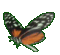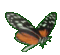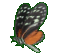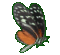Algebra Help - Relations & Ordered Pairs Data Help - Game Tips:

- The relation, Distance = Speed X Time represents a large set of data.
- As an example, if Speed = 4 m/s, then the relationship is Distance = (4)(Time) and the related data
can be described as a set of ordered pairs of the form (T,D).
Examples for (T,D) include the ordered pairs (3,12), (5,20), (10,40) where D=4T in each case.

The order of T & D in (T,D) is crucial. The D comes after the T because D is the outcome from D=4T.

- The relation y=mx also represents a large set of data.
- If, for example, m = 2, then the relationship is y = 2x and the related data
can be described as a set of ordered pairs of the form (x,y),
where (x,y) includes ordered pairs such as (3,6), (5,10), (12,24), since y=2x in each case.

- Your Game Score is reduced by the number of butterfly hits.

- To slow the game speed repeat tap/click on the word Slider.
- To increase the game speed repeat tap/click on the word Math.
- Speed can also be adjusted with a keyboard's - and + keys.

- Refresh/Reload the web page to restart the game.